### Home > MC1 > Chapter 3 > Lesson 3.4.2 > Problem3-106

3-106.
1. .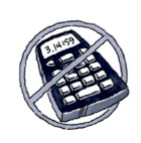Find each of the following products without a calculator. Draw a diagram or use words to explain how you know your product makes sense. Homework Help ✎

1. 6(−3)

2. −6(3)

3. −8(−3)

4. −8(0)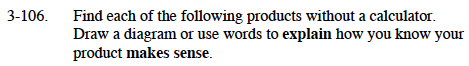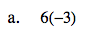By definition, multiplication is a shortcut for repeated addition. Now think of what it's like to multiply negative numbers. Can you put this in your own words?

The product is −18. Don't forget to explain why this makes sense!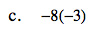Claire found the product of this expression and then explained that multiplying two negative numbers is like removing groups of negatives. Can you think of another way to explain this? Try drawing a diagram or putting Claire's idea into your own words.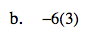It may help to think of multiplying a positive and negative number as removing groups of a positive number. For example, here you are removing groups of three, six times. Can you represent this in a diagram?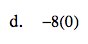Zero multiplied by any number will always be zero. Can you explain why?